Sample Paper Mathematics

# Mathematics Unsolved Sample Paper Solutions ICSE Class 10

## Section-A

(i) (c) ₹ 1,050

Explanation :

Let the price of the article be ₹ x.

So, x + GST = 1,239

x + 18% of x = 1,239

x+18x/100=1,239

118x/100=1,239

⇒ x = ₹ 1,050

(ii) (b) –2 √ 6
Explanation :

√2/3 is a solution of the equation

So, 3 ×(√2/3)2 + m × √(2/3)+2=0
3 ×2/3 + m × √(2/3)+2=0
m × √(2/3)=-4
m =-4√3/√2=0

m =-2√6

(iii) (c) 1/3

Explanation :
p(x) = x3 – ax2 + 2x + a – 1 (x – a) is a factor of p(x) a is the zero of p(x) p(x = a) = 0 ⇒ (a3) – a(a2) + 2a + a – 1 = 0
⇒ a3 – a3 + 3a – 1 = 0

⇒ 3a – 1 = 0

⇒ a = 1/3Explanation :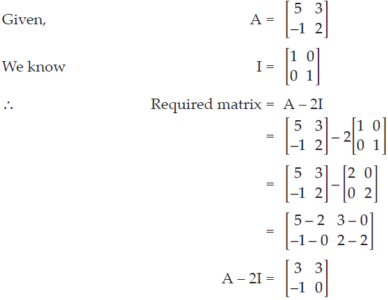(v) (c) 945

Explanation :

List of first 14 natural numbers, where each number is divisible by 9 is :
9, 18, 27, .................. .

Clearly, this list forms an A. P.

First term of A.P. (a) = 9

Common difference (d) = 9

We know,

Sn = n/2 [2a + (n – 1)d]
Sn = 14/2 [2×9 + (14 – 1)9]

= 7[18 + 117]

= 945

Required sum = 945

(vi) (d) (2, – 1)

Explanation :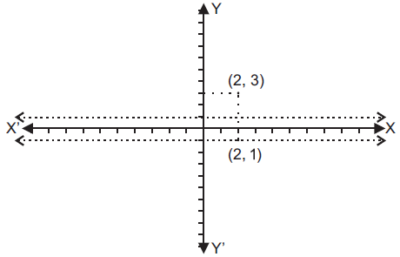(vii) (b) 6 cm

Explanation :

In ΔADE and DABC

∠ADE = ∠ABC  [Corresponding angles]

∠A = ∠A [Common angle]

∠ADE ~ ∠ABC [AA similarity rule]

⇒ 2/6=AE/9

⇒ AE = 3 cm

CE = AC – AE

= 9 – 3 = 6 cm.

(viii) (b) 2.5

Explanation :

Inequality 7 – 3x (-1/2)

⇒  – 3x (-1/2) - 7

⇒ – 3x (-15/2)

⇒ x ≤ 15/6

⇒ x ≤ 2.5

Greatest value of x = 2.5

(ix) (d) 8

Explanation :

No. of possible outcomes n(S) = n(HHH, HHT, HTH, HTT, THH, THT, TTH, TTT)
= 8.

(x) (b) 48π cm2

Explanation :
Let r = 2a and h = 3a Given Volume = πr2h = 96π cm3 ⇒ π × (2a)2 × 3a = 96π ⇒ 12a3 = 96 ⇒ a3 = 8 ⇒ a = 2 r = 2 × 2 = 4 cm and h = 2 × 3 = 6 cm Hence,   Curved surface area = 2πrh = 2 ×π × 4 × 6 = 48π cm2.

(xi) (d) 2

Explanation :and x – 2y = 6

⇒ x – 2(–2) = 6

⇒ x + 4 = 6

⇒ x = 6 – 4 = 2

(xii) (b) y = 2x

Explanation :

Let the equation be y = mx + c

As the slope is 2, y = 2x + c

as it is passing through (1,2), substituting this in the given equation

2 = 2 × 1 + c

⇒ c = 0 Hence, the equation is y = 2x

(xiii) (b) ∠A = ∠B

Explanation :

The angles at the circumference subtended by the same arc are equal.

(xiv) (c) n(n+1)/√2

Explanation :

Given: series is, √2+√8+√18+√32+.........

⇒ √2+2√2+3√2+4√2+......+n√2

⇒√2(1+2+3+4+.....+n)

We know that,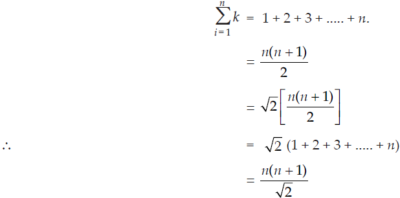We know that,

(xv) (c) Mode

Explanation :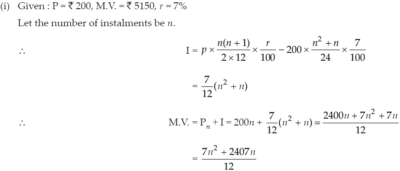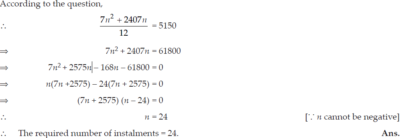(iii) (cosec θ – sin θ)(sec θ – cos θ) (tan θ + cot θ) = 1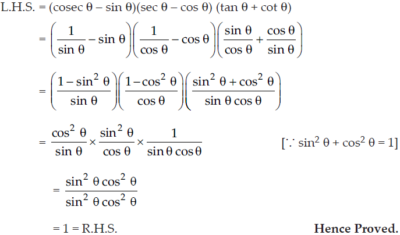(i) For cylinder 1 :

Diameter = 24 cm

Radius, R = 24/2 = 12 cm

Height, H = 220 cm

For cylinder 2 :

Radius, r = 8 cm

Height, h = 60 cm

Total volume of iron in two cylinders = πR2H + πr2h

= π(144 × 220 + 64 × 60) cm3

= 3.14 × 35520 = 111532.8 cm3

Total mass of iron = 111532.8 × 8 g

=111532.8 ×8/1000 kg

892.26 kg (approximately). Ans.

(ii) For Point P :

(x1, y1) = A(– 2, 6), (x2, y2) = B(3, – 4) and m1 : m2 = 2 : 3.(iii) (i) A (3, 5), B (– 2, – 4) are plotted on graph.(iv) AA′BB′ is an isosceles trapezium.

(v) Two invariant points under reflection in X-axis are C (3, 0) and D (– 2, 0).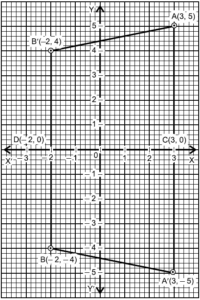## Section-B

(i) When the product is sold from Agra to Kanpur (intra - state transaction) :
For dealer in Agra :

S.P = ₹ 20,000

CGST = 9% of ₹ 20,000 = ₹ 1,800

SGST = 9% of ₹ 20,000 = ₹1,800

When product is sold from Kanpur to Jaipur (inter- state transaction):
For the dealer in Kanpur :

Input tax credit = ₹1,800 + ₹ 1,800 = ₹ 3,600

C.P = ₹ 20,000 and Profit = ₹ 5,000

S.P = ₹ 20,000 + ₹ 5,000 = ₹ 25,000

IGST = 18% of 25,000 = ₹ 4,500

Net GST paid by the dealer at Kanpur

= Output GST – Input GST

= 4,500 – 3,600 = ₹ 900

The cost of goods/services at Jaipur

= S.P in Kanpur + IGST

= 25,000 + 18% of 25,000

= 25,000 + 4,500 = ₹ 29,500

IGST = 18% of 25,000 = ₹ 4,500

= Output GST – Input GST

= 4,500 – 3,600 = ₹ 900

The cost of goods/services at Jaipur

= S.P in Kanpur + IGST

= 25,000 + 18% of 25,000

= 25,000 + 4,500 = ₹ 29,500

(ii) Given equation is,

(k + 1)x2 – 4kx + 9 = 0

Here, a = (k + 1), b = – 4k, c = 9

For real and equal roots,

D = b2 – 4ac = 0
⇒ (– 4k)2 – 4(k + 1)9 = 0
⇒ 16k2 – 36(k + 1) = 0

16k2 – 36k – 36 = 0

Dividing both sides by 4, we get

4k2 – 9k – 9 = 0
⇒ 4k2 – 12k + 3k – 9 = 0

⇒ 4k(k – 3) + 3(k – 3) = 0

⇒ (k – 3)(4k + 3) = 0

k – 3 = 0 4k + 3 = 0

k = 3   k = − 3/4

For k = 3, the equation will be

4x2 – 12x + 9 = 0
⇒ 4x2 – 6x – 6x + 9 = 0

⇒ 2x(2x – 3) – 3(2x – 3) = 0

⇒ (2x – 3)2 = 0

⇒ x = 3/2, 3/2

For k = – 3/4 , the equation will be

(-3+1/4)x2-4(-3/4)x+9=0
⇒ 1x2/4 + 3x + 9 = 0
⇒ x2 + 12x + 36 = 0
⇒ x2 + 6x + 6x + 36 = 0

⇒ x(x + 6) + 6(x + 6) = 0

⇒ (x + 6)2 = 0

⇒ x = – 6, – 6.

Hence, x = 3 /2 , − 6.

(iii)

 Class Interval Frequency 0 – 5 2 5 – 10 7 10 – 15 18 20 – 25 8 25 – 30 5 15 – 20 10

∴ Mode = 13 (using histogram).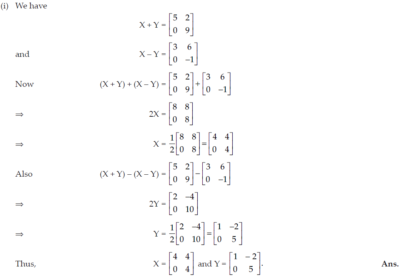(ii) Given, ∠SRT = 65° and SP is tangent

∠TSR = 90° [Angle between the radius and tangent]

∴ ∠SRT + ∠STR = ∠TSR = 90°

⇒ ∠STR = ∠TSR – ∠SRT

∠STR = x° = 90° – 65° = 25°

∠y° = 2∠x°

[Angle subtended at the centre is double that of the angle subtended by the arc at same circle]∴ ∠y° = 2 × 25° = 50°

and ∠SPO = ∠OSP – ∠SOP

∴ ∠SPO = ∠z = 90° – 50° = 40°

Hence, ∠x = 25°, ∠y = 50° and ∠z = 40°.  Ans.

(iii) Given expression is 2x3 – x2 – px – 2 and x – 2 is the factor.

(a) Put x – 2 = 0 ⇒ x = 2 in expression, we get

2(2)3 – (2)2 – p(2) – 2 = 0

⇒ 16 – 4 – 2p – 2 = 0

⇒ 10 – 2p = 0

⇒ p = 5  Ans.

(b) Putting the value of p, the expression becomes 2x3 – x2 – 5x – 2.

Dividing the expression by (x – 2),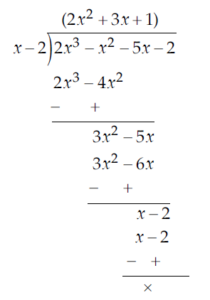∴ 2x3 – x2 – 5x – 2 = (x – 2)(2x2 + 3x + 1)

= (x – 2) {2x2 + (2 + 1) x + 1}

= (x – 2) (2x2 + 2x + x + 1)

= (x – 2) {2x (x + 1) +1 (x + 1)}

= (x – 2) (2x + 1) (x + 1) Ans.

(i) Given, A(7, – 3) and B(1, 9).

(a) Slope of AB = y2-y1/x2-x1

= 9-(-3) /1-7

=12/-6

=-2

(b) Slope of perpendicular bisector of AB (m) = -1/(-2)=1/2

Mid-point of AB =((x1+x2)/2,(y1+y2)/2)

=((7+1)2,(-3+9)/2)=(4,3)

Let the equation of required line be

y – y1 = m(x – x1)

⇒ y – 3 = 1/2 (x – 4)

⇒ 2y – 6 = x – 4

⇒ x – 2y – 4 + 6 = 0

⇒ x – 2y + 2 = 0, which is the required line. Ans.

(c) Since (– 2, p) lies on x – 2y + 2 = 0

Putting x = 2, y = p, we get

– 2 – 2p + 2 = 0

⇒ 2p = 0

⇒ p = 0 Ans.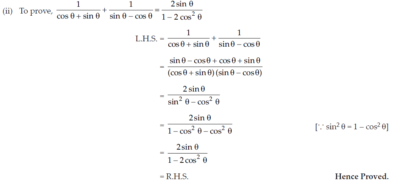(iii) Let the first term of A.P. be a and the common difference be d.
Given,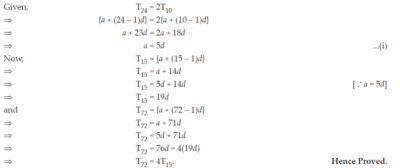(i) After removing king, queen and jack of clubs, 49 cards are left in the deck.

∴ Total number of possible outcomes = 49

(a) There are 3 kings left in the deck.

∴ Number of favourable outcomes = 3

∴ P (a king) = 3/49. Ans.

(b) After removing king, queen and jack of clubs, number of clubs cards left = 13 – 3 = 10.

∴ No. of favourable outcomes = 10

∴ P (a club) = 10/49. Ans.

(c) There is only one ‘10’ of hearts

∴ No. of favourable outcomes = 1

∴ P (a ‘10’ of hearts) = 1/49. Ans.

(ii) According to the question,

2/3 (Volume of hemisphere) = Volume of cone

2/3(2 πr3/3) = -1πr2h/3
4/9 (3.5)3=1/3(3.5)2.h

h =4 ×  3.5 × 3.5 × 3.5 × 3/3.5 × 3.5 × 9

42.0/914m/3 = 4.67 m

Slant height of cone, l = √r2+h2
=√(3.5)2+(4.67)2

35m/6

Now,  Surface area of buoy = Surface area of right cone + Surface area of hemisphere

= πrl + 2πr2

= pr(l + 2r)

22/7 × 3.5 ((35/6)+2 × 3.5)

= 11 × (5.83 + 7)

= 11 × 12.83

= 141.13 m2 Ans.

(iii) Given, AB is a diameter.

and  ∠BAC = 40°.In ΔABC

∴ ∠ABC + ∠ACB + ∠BAC = 180° [Sum of angles in a D is 180°]

⇒  ∠ABC + 90° + 40° = 180°

∠ABC = 180° – 130° = 50°

Now, ∠ACT = ∠ABC [Angles in alternate segment]

⇒ ∠ACT = 50°. Ans.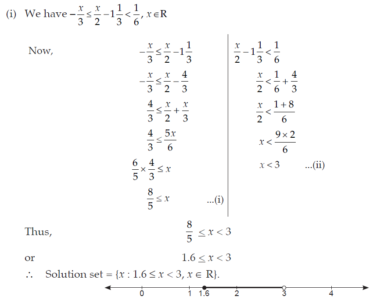(i)

 Marks : Less than Classes Mid Values (x) No. of students (c.f.) Frequency (f) fx 10 0 – 10 5 2 2 10 20 10 – 20 15 7 5 75 30 20 – 30 25 15 8 200 40 30 – 40 35 18 3 105 50 40 – 50 45 20 2 90 Σf = 20 Σfx = 480

∴ Arithmetic mean = Σfx/Σf=480/20=24

(iii) Given, ∠ABC = ∠DAC = x (say)

AB = 8 cm, AC = 4 cm, AD = 5 cm.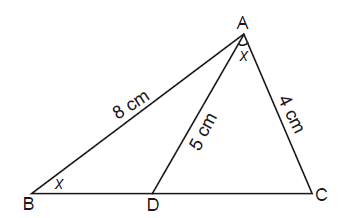(a) In ΔACD andΔBCA

∠ABC = ∠DAC [Given]

∠ACD = ∠BCA [Common]

∴ ΔACD ~ ΔBCA [AA axiom]

Hence ΔACD is similar to ΔBCA. Hence Proved.

(b) ΔACD ~ ΔBCA

∴ AC/BC=CD/CA=AD/BA  [Corresponding parts of similar triangles]

⇒  4/BC=CD/4=5/8

⇒ 4/BC = 5/8

⇒ BC = 8 x 4/5=32/5=6.4 CM

and CD/4 = 5/8

⇒ CD = 5 x 4 /8

⇒ CD = 2.5 cm. Ans.

(i) The original cost of each book = ₹ x.

Total cost = ₹ 960

No. of books purchased = 960/x

If the price was ₹ 8 less, the cost of each book = ₹ (x – 8)water.

No. of books purchased = 960/x -8

According to question,

(960/x-8)-(960/x) = 4

960(1/x-8)-(1/x) = 4

960 x (x-x+8)/x(x-8)=4

⇒ 960 × 8 = 4x (x – 8)

⇒ 4x2 – 32x = 7680
⇒ x2 – 8x – 1920 = 0
⇒ x2 – 48x + 40x – 1920 = 0

⇒ x (x – 48) + 40 (x – 48) = 0

⇒ (x – 48) (x + 40) = 0

⇒ x – 48 = 0  or   x + 40 = 0

⇒ x = 48 or x = – 40 (not possible)

x = 48

The original cost of each book = ₹ 48.

(ii)

 Marks No. of Students c.f. 0 – 10 5 5 10 – 20 11 16 20 – 30 10 26 30 – 40 20 46 50 – 60 37 111 60 – 70 40 151 70 – 80 29 180 80 – 90 14 194 90 – 100 6 200

∴ N = 200 [even]

(a) Median = Nth/2 value

= 100th value

= 57 marks (from ogive) Ans.

(b) No. of students scoring less than 40 marks = 45.(i) We have,

(x4+1)/2x2=17/8
(x4+1+2x2)/(x4+1-2x2)=17+8/17+8
⇒( (x2)2+2.x2.1+1)/( (x2)2-2.x2.1+1)=25/9
⇒ (x2 +1)2/(x2 -1)2 =25/9
⇒ (x2+1)/(x2 - 1) =5/3
⇒  5(x2 – 1) = 3(x2 + 1)
⇒ 5x2 – 5 = 3x2 + 3
⇒ 5x2 – 3x2 = 3 + 5
⇒  2x2 = 8
⇒ x2 = 4

⇒  x = ± 2. Ans.

(ii) Steps of construction :

(a) Draw DABC with each side 5 cm.

(b) Bisect ∠ABC and ∠ACB.

(c) Let the bisectors meet at O.

(d) Draw a perpendicular OD from O to BC.

(e) Taking OD as radius, draw the required incircle.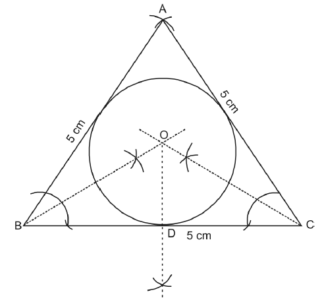(iii) Let the aeroplane be at position A and BC be the river. Drop a perpendicular from A on BC. Let it intersect BC at D.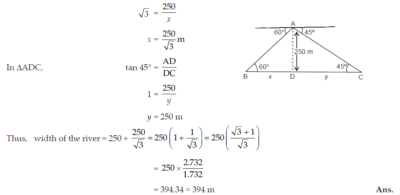#### ICSE 61 Sample Question Papers

All Subjects Combined for Class 10 Exam 2023

The dot mark field are mandatory, So please fill them in carefully
To download the Sample Paper (PDF File), Please fill & submit the form below.

Free Shipping on all
orderes over ₹ 1,000

Free 10-Day
Return Policy

15-Days Money-back
Guarantee

23,125 Products
Shipped this Month

Need Help?
+91-745 507 7222

Shopping cart